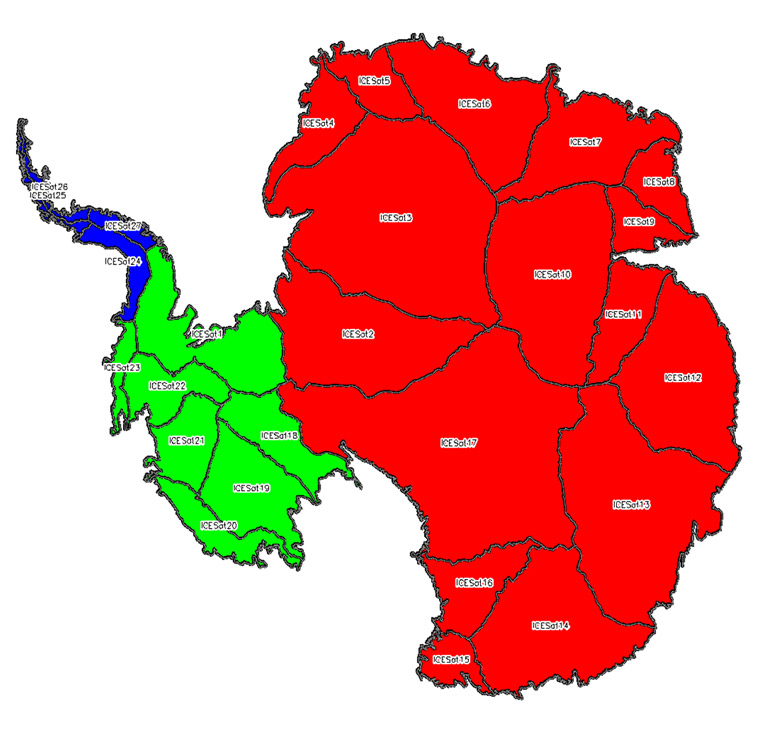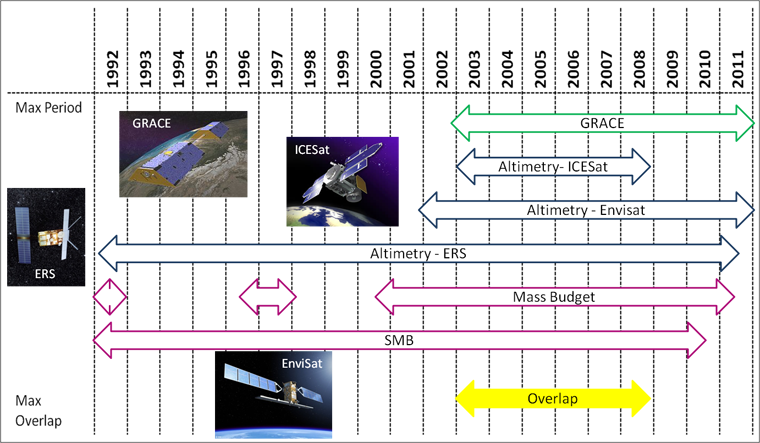# Experiments

Home > IMBIE 2012 > Experiments

The approach followed within IMBIE 2012 consisted of inter-comparison experiments to determine the level of agreement between estimates of mass balance derived from radar and laser altimetry, gravimetry and the input-output method. After this, the data were combined to produce a reconciled estimate of mass balance for each ice sheet region.

For the inter-comparison experiments we aimed to eliminate the impact of differing dataset coverage by using a common time period and standardised regions. We defined the common time period as 2003 to 2008, as this was the period of overlap when all satellite geodetic techniques were operating optimally (see figures below). The regions for which mass balance estimates were made included the Greenland, East Antarctic, West Antarctic and Antarctic Peninsula Ice Sheets. In Antarctica, standard geographical boundary definitions for the Antarctic Peninsula, East Antarctic and West Antarctic Ice Sheets were used that were based on the definition of Zwally et al., (2012) (see figures below).

For each of the techniques the data processing methodologies, ancillary datasets and corrections were investigated independently. In addition, different glacial isostatic adjustment and surface mass balance models were investigated and their impacts on the mass balance estimates considered. In the case of techniques involving numerous research groups, (i.e. laser altimetry, gravimetry, glacial isostatic adjustment and surface mass balance) inter-comparisons of results from the different research groups were conducted. The arithmetic means of ice sheet mass imbalance estimates from each technique for the standard time period and each region were computed and compared in order to assess the extent to which the results agreed.

To produce the reconciled estimate of ice sheet mass balance, we computed the average rate of mass change derived from each of the geodetic techniques, within the various regions of interest and over the maximum time periods for which geodetic mass rates could be derived (e.g. for radar altimetry this was for a 19 year time period). We then calculated the linear average of the individual mass balance values to arrive at reconciled values, and integrated these data to form a time series of cumulative mass change within each of the four ice sheet regions. A measure of the collective uncertainty of the estimates was taken to be the standard error of the uncertainty estimates from the independent techniques.The division of the Antarctic Ice Sheet as defined by Zwally et al., (2012). The Antarctic Peninsula, West and East Antarctic Ice Sheets are shown in blue, green and red respectively. The black lines delineate 27 different drainage sectors.The time coverage and overlapping period of the datasets used in IMBIE 2012.

,